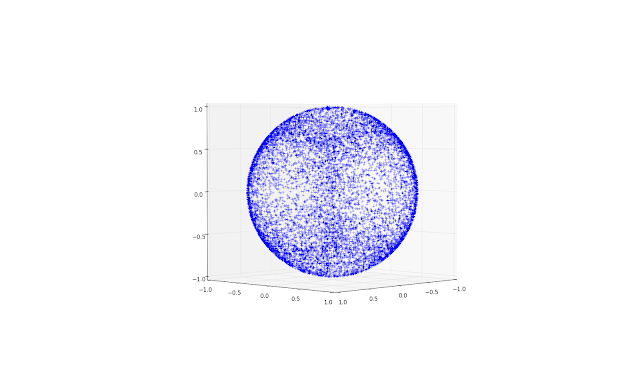## Saturday, April 8, 2017

### Video games to statistical mechanics

A friend was writing a computer game in his spare time and wanted to speckle a sphere with texture uniformly over its surface. Idly, he asked our team how to do this. We came up with some naive ideas like taking the x,y and z co-ordinates from a uniform sample and normalizing them to make a vector or length r, the radius of the sphere. In Python, this would be:

import random, math, pylab, mpl_toolkits.mplot3d

x_list, y_list, z_list = [],[],[]
nsamples = 10000
for sample in xrange(nsamples):
x, y, z = random.uniform(-1.0, 1.0), random.uniform(-1.0, 1.0), random.uniform(-1.0, 1.0)
radius = math.sqrt(x ** 2 + y ** 2 + z ** 2)
fig = pylab.figure()
ax = fig.gca(projection='3d')
ax.set_aspect('equal')
pylab.plot(x_list, y_list, z_list, '+')
pylab.show()

but this is not quite uniformly distributed over the sphere. It produces a sphere like this:Sphere with Cartesian co-ordinates taken from a uniform sample and then normalized

Using polar co-ordinates and uniformly sampling over 2π doesn't make things better either. This:

for sample in xrange(nsamples):
phi, theta = random.uniform(0, 2.0) * math.pi, random.uniform(0, 2.0) * math.pi
x_list.append(math.cos(phi) * math.cos(theta))
y_list.append(math.cos(phi) * math.sin(theta))
z_list.append(math.sin(phi))

gives:Sphere with polar co-ordinates taken from a uniform sample
which is also clearly not evenly spread.

The solution involves Gaussian (a.k.a 'Normal') distributions, thus:

for sample in xrange(nsamples):
x, y, z = random.gauss(0.0, 1.0), random.gauss(0.0, 1.0), random.gauss(0.0, 1.0)
radius = math.sqrt(x ** 2 + y ** 2 + z ** 2)